# Modeling Engine Timing Using Triggered Subsystems

This example shows how to model a four-cylinder spark ignition internal combustion engine from the throttle to the crankshaft output. We used well-defined physical principles supplemented, where appropriate, with empirical relationships that describe the system's dynamic behavior without introducing unnecessary complexity.

### Analysis and Physics

This example describes the concepts and details surrounding the creation of engine models with emphasis on important Simulink® modeling techniques. The basic model uses the enhanced capabilities of Simulink to capture time-based events with high fidelity. Within this simulation, a triggered subsystem models the transfer of the air-fuel mixture from the intake manifold to the cylinders via discrete valve events. This takes place concurrently with the continuous-time processes of intake flow, torque generation and acceleration. A second model adds an additional triggered subsystem that provides closed-loop engine speed control via a throttle actuator. These models can be used as standalone engine simulations. Or, they can be used within a larger system model, such as an integrated vehicle and powertrain simulation, in the development of a traction control system.

This model is based on published results by Crossley and Cook (1991). It describes the simulation of a four-cylinder spark ignition internal combustion engine. The Crossley and Cook work also shows how a simulation based on this model was validated against dynamometer test data. The ensuing sections (listed below) analyze the key elements of the engine model that were identified by Crossley and Cook:

1. Throttle

2. Intake manifold

3. Mass flow rate

4. Compression stroke

5. Torque generation and acceleration

• Note: Additional components can be added to the model to provide greater accuracy in simulation and to more closely replicate the behavior of the system.

### Throttle

The first element of the model is the throttle body. The control input is the angle of the throttle plate. The rate at which the model introduces air into the intake manifold can be expressed as the product of two functions:

1. an empirical function of the throttle plate angle only

2. a function of the atmospheric and manifold pressures

In cases of lower manifold pressure (greater vacuum), the flow rate through the throttle body is sonic and is only a function of the throttle angle. This model accounts for this low pressure behavior with a switching condition in the compressibility equations shown in Equation 1.

Equation 1### Intake Manifold

The simulation models the intake manifold as a differential equation for the manifold pressure. The difference in the incoming and outgoing mass flow rates represents the net rate of change of air mass with respect to time. This quantity, according to the ideal gas law, is proportional to the time derivative of the manifold pressure (see Equation 2). Note that, unlike the model of Crossley and Cook (see also references 3 through 5), this model doesn't incorporate exhaust gas recirculation (EGR), although this can easily be added.

Equation 2### Intake Mass Flow Rate

The mass flow rate of air that the model pumps into the cylinders from the manifold is described in Equation 3 by an empirically derived equation. This mass rate is a function of the manifold pressure and the engine speed.

Equation 3To determine the total air charge pumped into the cylinders, the simulation integrates the mass flow rate from the intake manifold and samples it at the end of each intake stroke event. This determines the total air mass that is present in each cylinder after the intake stroke and before compression.

### Compression Stroke

In an inline four-cylinder four-stroke engine, 180 degrees of crankshaft revolution separate the ignition of each successive cylinder. This results in each cylinder firing on every other crank revolution. In this model, the intake, compression, combustion, and exhaust strokes occur simultaneously (at any given time, one cylinder is in each phase). To account for compression, the combustion of each intake charge is delayed by 180 degrees of crank rotation from the end of the intake stroke.

### Torque Generation and Acceleration

The final element of the simulation describes the torque developed by the engine. An empirical relationship dependent upon the mass of the air charge, the air/fuel mixture ratio, the spark advance, and the engine speed is used for the torque computation (see Equation 4).

Equation 4Calculate the engine angular acceleration using Equation 5

Equation 5### Open-Loop Model

We incorporated the model elements described above into an engine model using Simulink. The following sections describe the decisions we made for this implementation and the key Simulink elements used. This section shows how to implement a complex nonlinear engine model easily and quickly in Simulink environment. We developed this model in conjunction with Ken Butts, Ford Motor Company® (2).

Figure 1 shows the top level of the model. Note that, in general, the major blocks correspond to the high-level list of functions given in the model description in the preceding summary. Taking advantage of Simulink's hierarchical modeling capabilities, most of the blocks in Figure 1 are made up of smaller blocks. The following paragraphs describe these smaller blocks.

### Running the Simulation

Press the "Play" button on the model toolbar to run the simulation.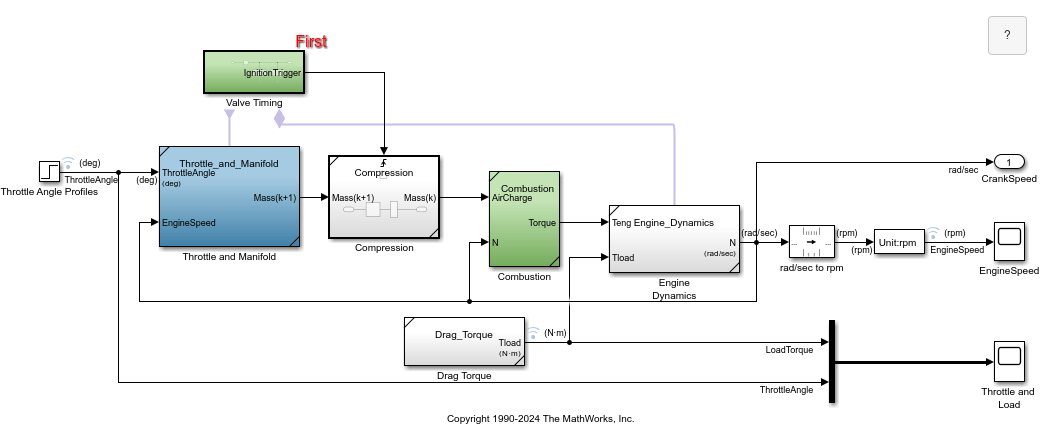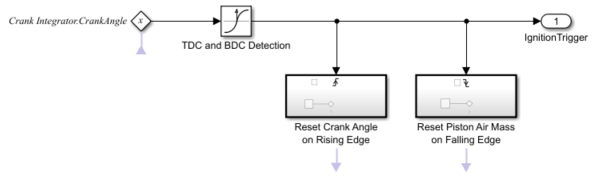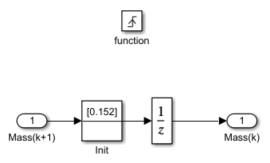Figure 1: The top level of the engine model and simulation results

• Note: The model logs relevant data to MATLAB workspace in a structure called sldemo_engine_output. Logged signals have a blue indicator. Read more about Signal Logging in Simulink documentation.

### Throttle/Manifold

In the model, double click on the 'Throttle & Intake Manifold' subsystem to open it. It contains two other subsystems - the 'Throttle' and the 'Intake Manifold' subsystems. Open the 'Throttle' and 'Intake Manifold' to see their components.Figure 2: The 'Throttle' and 'Intake Manifold' subsystems

Simulink models for the throttle and intake manifold subsystems are shown in Figure 2. The throttle valve behaves in a nonlinear manner and is modeled as a subsystem with three inputs. Simulink implements the individual equations, given in Equation 1, as function blocks. These provide a convenient way to describe a nonlinear equation of several variables. A 'Switch' block determines whether the flow is sonic by comparing the pressure ratio to its switch threshold, which is set at one half (Equation 1). In the sonic regime, the flow rate is a function of the throttle position only. The direction of flow is from the higher to lower pressure, as determined by the Sign block. With this in mind, the 'Min' block ensures that the pressure ratio is always unity or less.

The differential equation from Equation 2 models the intake manifold pressure. A Simulink function block computes the mass flow rate into the cylinder, a function of manifold pressure and engine speed (see Equation 3).

### Intake and Compression

An integrator accumulates the cylinder mass air flow in the 'Intake' block (located inside the 'Throttle & Manifold' subsystem). The 'Valve Timing' block issues pulses that correspond to specific rotational positions in order to manage the intake and compression timing. Valve events occur each cam rotation, or every 180 degrees of crankshaft rotation. Each event triggers a single execution of the 'Compression' subsystem. The output of the trigger block within the 'Compression' subsystem then feeds back to reset the Intake integrator. In this way, although both triggers conceptually occur at the same instant in time, the integrator output is processed by the 'Compression' block immediately prior to being reset. Functionally, the 'Compression' subsystem uses a 'Unit Delay' block to insert 180 degrees (one event period) of delay between the intake and combustion of each air charge.

Consider a complete four-stroke cycle for one cylinder. During the intake stroke, the 'Intake' block integrates the mass flow rate from the manifold. After 180 degrees of crank rotation, the intake valve closes and the 'Unit Delay' block in the 'Compression' subsystem samples the integrator state. This value, the accumulated mass charge, is available at the output of the 'Compression' subsystem 180 degrees later for use in combustion. During the combustion stroke, the crank accelerates due to the generated torque. The final 180 degrees, the exhaust stroke, ends with a reset of the Intake integrator, prepared for the next complete 720 degrees cycle of this particular cylinder.

For four cylinders, we could use four 'Intake' blocks, four 'Compression' subsystems, etc., but each would be idle 75% of the time. We've made the implementation more efficient by performing the tasks of all four cylinders with one set of blocks. This is possible because, at the level of detail we've modeled, each function applies to only one cylinder at a time.

### Combustion

Engine torque is a function of four variables. The model uses a 'Mux' block to combine these variables into a vector that provides input to the 'Torque Gen' block. A function block computes the engine torque (described empirically in Equation 4). The torque which loads the engine, computed by step functions in the Drag Torque block, is subtracted in the Engine Dynamics subsystem. The difference divided by the inertia yields the acceleration, which is integrated to arrive at the engine crankshaft speed.

### Plotting Simulation Results

We used the following default inputs for the simulation:Try adjusting the throttle to compensate for the load torque. Figure 3 shows the simulated engine speed, the throttle commands which drive the simulation, and the load torque which disturbs it.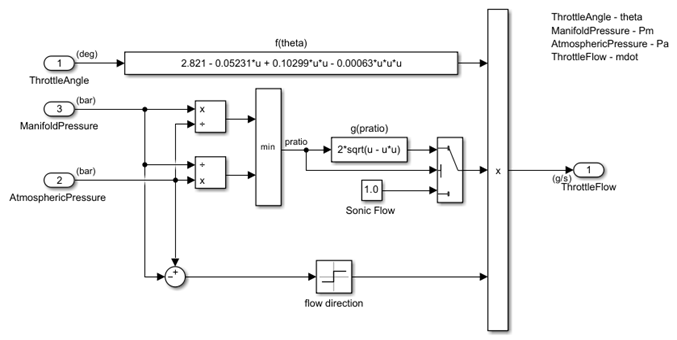Figure 3a: Open-loop simulation inputs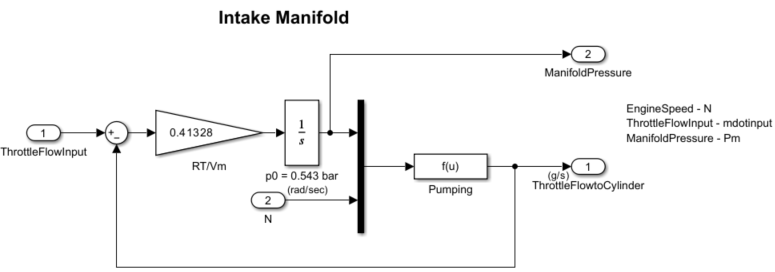Figure 3b: Open-loops simulation results

### Closing Model

Close the model. Clear generated data.

### Conclusions

The ability to model nonlinear, complex systems, such as the engine model described here, is one of Simulink's key features. The power of the simulation is evident in the presentation of the models above. Simulink retains model fidelity, including precisely timed cylinder intake events, which is critical in creating a model of this type. The basic engine model shows the flexibility of Simulink.

### References

 P.R. Crossley and J.A. Cook, IEEE® International Conference 'Control 91', Conference Publication 332, vol. 2, pp. 921-925, 25-28 March, 1991, Edinburgh, U.K.

 The Simulink Model. Developed by Ken Butts, Ford Motor Company. Modified by Paul Barnard, Ted Liefeld and Stan Quinn, MathWorks®, 1994-7.

 J. J. Moskwa and J. K. Hedrick, "Automotive Engine Modeling for Real Time Control Application," Proc.1987 ACC, pp. 341-346.

 B. K. Powell and J. A. Cook, "Nonlinear Low Frequency Phenomenological Engine Modeling and Analysis," Proc. 1987 ACC, pp. 332-340.

 R. W. Weeks and J. J. Moskwa, "Automotive Engine Modeling for Real-Time Control Using Matlab/Simulink," 1995 SAE Intl. Cong. paper 950417.